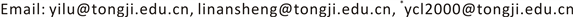1. 引言

2. 多悬臂梁–流体的模态耦合2.1. 双悬悬臂梁–流体的模态耦合

The first two frequencies of cantilever beam with one side infinite wate

327.32165.97
3.629.91181.62
4.232.30195.93
4.834.53209.15

ANSYS模拟得到的曲线与文献  中理论解法得到的结果一致，结果表明：两个梁组成的一个系统，其系统某一阶自然频率有两个，当梁间距较小时，由于液体的耦合作用，系统的两个频率发生了很大变化，说明两个梁通过液体耦合发生了相互作用；随着梁间距增大，2a/h大于3时，系统的两个频率趋于直线，与单个梁与无穷水体作用的自然频率相同，说明梁间的耦合作用趋于零。

2.2. 三悬臂梁–流体结构模态影响

3. 耦合自由振动的拍现象

3.1. 双梁–液体系统拍振现象

{ u a ( z , t ) = ∑ i = 1 ∞ q i Y a i ( z ) ⋅ cos ( ω i ⋅ t + φ i ) u b ( z , t ) = ∑ i = 1 ∞ q i Y b i ( z ) ⋅ cos ( ω i ⋅ t + φ i ) (1)

{ u a ( z , t ) = q 1 Y a 1 ( z ) ⋅ cos ( ω 1 ( 1 ) ⋅ t + φ 1 ) + q 2 Y a 2 ( z ) ⋅ cos ( ω 1 ( 2 ) ⋅ t + φ 2 ) u b ( z , t ) = q 1 Y b 1 ( z ) ⋅ cos ( ω 1 ( 1 ) ⋅ t + φ 1 ) + q 2 Y b 2 ( z ) ⋅ cos ( ω 1 ( 2 ) ⋅ t + φ 2 ) (2)

u a ( z , 0 ) = Y ( z ) ; d u a d t | t = 0 = u b | t = 0 = d u b d t | t = 0 = 0 (3)

{ u a = Y ( z ) cos ( ω 1 ( 2 ) − ω 1 ( 1 ) 2 ⋅ t ) cos ( ω 1 ( 2 ) + ω 1 ( 1 ) 2 ⋅ t ) u b = − Y ( z ) sin ( ω 1 ( 2 ) − ω 1 ( 1 ) 2 ⋅ t ) sin ( ω 1 ( 2 ) + ω 1 ( 1 ) 2 ⋅ t ) (4)

3.2. 三梁–液体系统拍振现象

4. 结论

1) 由于流固耦合作用的存在，悬臂梁之间存在相互作用，这种相互作用影响了耦联结构的湿模态，且这种影响随着梁间液体宽度的增加而减弱。

2) 多悬臂梁–流体耦联系统拍振现象是由梁间相互作用产生的模态频率差导致，由于不同模态对不同梁位移函数贡献不同，不同梁的拍现象频率及振幅一般不同。